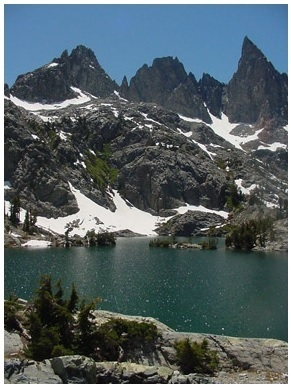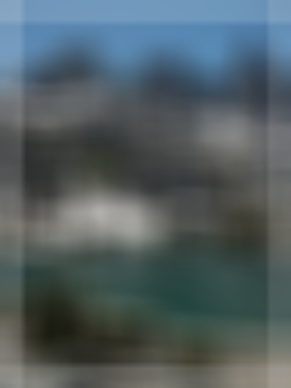# OpenCV - Box Filter

The Box Filter operation is similar to the averaging blur operation; it applies a bilateral image to a filter. Here, you can choose whether the box should be normalized or not.

You can perform this operation on an image using the boxFilter() method of the imgproc class. Following is the syntax of this method −

```boxFilter(src, dst, ddepth, ksize, anchor, normalize, borderType)
```

This method accepts the following parameters −

• src − A Mat object representing the source (input image) for this operation.

• dst − A Mat object representing the destination (output image) for this operation.

• ddepth − A variable of the type integer representing the depth of the output image.

• ksize − A Size object representing the size of the blurring kernel.

• anchor − A variable of the type integer representing the anchor point.

• Normalize − A variable of the type boolean specifying weather the kernel should be normalized.

• borderType − An integer object representing the type of the border used.

## Example

The following program demonstrates how to perform the Box Filter operation on an image.

```import org.opencv.core.Core;
import org.opencv.core.Mat;
import org.opencv.core.Point;
import org.opencv.core.Size;
import org.opencv.imgcodecs.Imgcodecs;
import org.opencv.imgproc.Imgproc;

public class BoxFilterTest {
public static void main( String[] args ) {

// Reading the Image from the file and storing it in to a Matrix object
String file = "E:/OpenCV/chap11/filter_input.jpg";

// Creating an empty matrix to store the result
Mat dst = new Mat();

// Creating the objects for Size and Point
Size size = new Size(45, 45);
Point point = Point(-1, -1);

// Applying Box Filter effect on the Image
Imgproc.boxFilter(src, dst, 50, size, point, true, Core.BORDER_DEFAULT);

// Writing the image
Imgcodecs.imwrite("E:/OpenCV/chap11/boxfilterjpg", dst);

System.out.println("Image Processed");
}
}
```

Assume that following is the input image filter_input.jpg specified in the above program.## Output

On executing the program, you will get the following output −

```Image Processed
```

If you open the specified path, you can observe the output image as follows −